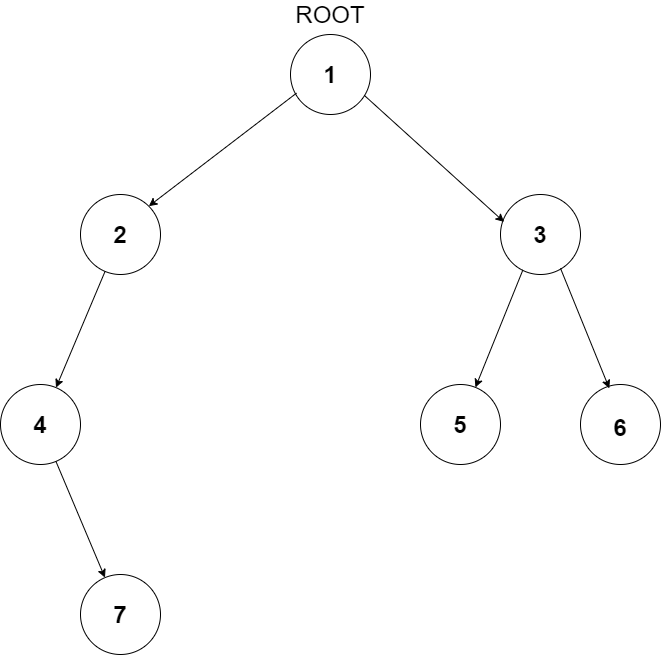# Subtree of Another Tree

Posted: 22 Nov, 2020
Difficulty: Easy

## PROBLEM STATEMENT

#### A subtree of a tree T is a tree S consisting of a node in T and all of its descendants in T. The subtree corresponding to the root node is the entire tree; the subtree corresponding to any other node is called a proper subtree.

##### Input Format:
``````The first line of input contains an integer ‘T’ representing the number of test cases. Then the test cases follow.

The first line of each test case contains elements of the first tree in the level order form. The line consists of values of nodes separated by a single space. In case a node is null, we take -1 in its place.

The second line of each test case contains elements of the second tree in the level order form. The line consists of values of nodes separated by a single space. In case a node is null, we take -1 in its place.

For example, the input for the tree depicted in the below image would be:
````````````1
2 3
4 -1 5 6
-1 7 -1 -1 -1 -1
-1 -1
``````

#### Explanation:

``````Level 1:
The root node of the tree is 1

Level 2:
Left child of 1 = 2
Right child of 1 = 3

Level 3:
Left child of 2 = 4
Right child of 2 = null (-1)
Left child of 3 = 5
Right child of 3 = 6

Level 4:
Left child of 4 = null (-1)
Right child of 4 = 7
Left child of 5 = null (-1)
Right child of 5 = null (-1)
Left child of 6 = null (-1)
Right child of 6 = null (-1)

Level 5:
Left child of 7 = null (-1)
Right child of 7 = null (-1)

The first not-null node(of the previous level) is treated as the parent of the first two nodes of the current level. The second not-null node (of the previous level) is treated as the parent node for the next two nodes of the current level and so on.
The input ends when all nodes at the last level are null(-1).
``````
##### Note:
``````The above format was just to provide clarity on how the input is formed for a given tree.
The sequence will be put together in a single line separated by a single space. Hence, for the above-depicted tree, the input will be given as:

1 2 3 4 -1 5 6 -1 7 -1 -1 -1 -1 -1 -1
``````
##### Output Format:
``````For each test case, the only line of output prints true if tree S is a subtree of the tree T else prints false.

The output for each test case is in a separate line.
``````
##### Note:
``````You do not need to print anything; it has already been taken care of.
``````
##### Constraints:
``````1 <= T <= 100
1 <= N, M <= 1000
0 <= data <= 10^6 and data != -1

Where ‘T’ is the number of test cases, ‘N’ and ‘M’ are the number of nodes in the given binary trees’, and “data” is the value of the binary tree node.

Time Limit: 1 sec.
``````Approach 1

Traverse the tree T in preorder fashion and treat every node of the given tree T as the root, treat it as a subtree and compare the corresponding subtree with the given subtree S for equality. For checking the equality, we can compare all the nodes of the two subtrees.

So the basic idea is to traverse over the given tree T and treat every node as the root of the subtree currently being considered and compare the corresponding subtree with the given subtree S for equality. To check the equality of the two subtrees, we make use of areIdentical(t,s) function, which takes t and s, which are roots of the two subtrees to be compared as the inputs and returns “true” or “false” depending on whether the two are equal or not. It compares all the nodes of the two subtrees for equality. Firstly, it checks the roots of the two trees for equality and then calls itself recursively for the left subtree and the right subtree.